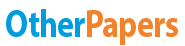# Breeden Electronics by Kaushiki Goswami

Essay by   •  August 29, 2019  •  Case Study  •  2,434 Words (10 Pages)  •  2,282 Views

## Essay Preview: Breeden Electronics by Kaushiki Goswami

Report this essay
Page 1 of 10

Assignment 2 Answers: Breeden Electronics by Kaushiki Goswami

1. What would break-even sales volume be, assuming a ratio of two RC1s sold for each RC2 sold?
2. What level of sales would provide the \$210,000 profit target for the year, as specified by the parent company? (Assume that Breeden Electronics sells all they produce.)
3. What would be the manufacturing cost per unit if they made and sold only 8,000 RC1 units and 4,000 RC2 units per month? In that case, what would the profit be?
4. What would profit be if they sold 8,000 RC1 units and 4,000 RC2 units (as in question 3) but produced 10,000 RC1 units and 5,000 RC2 units, putting the unsold units in finished goods inventory? Explain the difference in your answers to questions 3 and 4.
5. Recompute product costs for RC1 and RC2 by completing the work Marlene Baer began.

Break-even point is the point where there are zero profit or sales equal’s costs. In this case, we assume the same thing as above, that is, the manufacturing costs per unit are \$ 16 for RC 1 and \$ 19 for RC 2. Also assuming that RC 2’s are half that of RC 1, that is RC2= ½ RC1. The selling and admin expenses amount to \$ 480,000 for the year. This gives us the break-even sales of 80,000 units of RC 1 and 40,000 units of RC 2 per year or 6667 units of RC1 and 3333 units of RC2 per month.
Calculation: The selling price for RC 1 is \$ 20 per unit, and RC 2’s selling price is \$ 23 per unit. In this case, we assume that the manufacturing cost per unit is the same, that is, \$ 16 per unit for RC 1 and \$ 19 per unit for RC 2. This means that per unit selling price net of manufacturing costs is \$ 4 per unit for RC 1, as well as, RC 2. The monthly fixed selling and administrative expenses of \$ 40,000 translate into \$ 480,000 per annum. We also assume that the product mix would remain the same that is 2 RC 1’s for each RC 2. After calculation, we estimate that in order to get a yearly profit of \$ 210,000, Breeden security must sell 115,000 units of RC 1 and 57,500 units of RC 2 per year or 9,583 units of RC 1 and 4,792 units of RC 2 per month.
Calculation:
(20-16) RC1 + (23-19) RC2 = (40,000 x 12) + 210,000
Assuming, RC2 = RC1/2
4 RC1 + 2RC1 = 690,000
RC1 = 115,000 units
RC2 = 57,500 units.

20 RC1 + 23RC2 = (40,000 x 12) + 16RC1 +19RC2
Substituting RC2 with RC1/2,
31.5RC1 = 480,000 + 25.5RC1
RC1= 80,000 units per annum
RC2= 40,000 units per annum.

To calculate the breakeven volume; it is assumed that 2 units of RC1 are produced for every unit of RC2. This is assumed because RC2 is more expensive then RC1. Moreover, the demand for RC 2 is higher than the demand for RC1. For the calculation of breakeven volume, the costs are first divided into variable and fixed costs. The material (parts) and direct labor cost are both variable costs. The values for these costs available in month budget are divided by the unit volume and added together in order to get per unit variable cost. This variable cost is subtracted from the selling price per unit to calculate the contribution per unit. The contribution per unit is \$11 for RC1 and \$12.4 for RC2.

A combined contribution is calculated treating two RC1 and one RC2 as one unit and adding the contribution per unit of each unit. This combined contribution is \$34.4.
The remaining costs like manufacturing overheads and selling and administrative expenses are fixed costs. The total monthly fixed cost is \$152,000. This monthly cost is multiplied by twelve to get the total fixed cost of 2008. The fixed cost is divided by the combined contribution to get the breakeven volume of two RC1 and one RC2.
The table shows that in order to breakeven, the company needs to produce and sell 8,837 units and 4,419 units of RC1 and RC2, respectively. The margin of safety is 1, 163 units of RC1 and 581 units of RC2. This means that the company would need to produce and sell around 106,047 units of RC1 and 53,023 units of RC2 in the entire year of 2008 in order to cover all its manufacturing, selling and administrative costs.
BS has a contract with a large producer of motorized garage door. This contract ensures a minimum of 100,000 RC1 control units being ordered and supplied each. The minimum amount is a little less than the breakeven volume, but it can be assumed that the producer of these garage doors would order more than 100,000 units of RC1. Thus, the expected sale of 120,000 units of RC1 seems reasonable. Similarly, RC2 is often sold through the mail-order catalogue. For RC2, a sale of 60,000 is expected which is half the estimated sale of RC2 because RC2 is more expensive and is not sold through contract with a company.

...

...